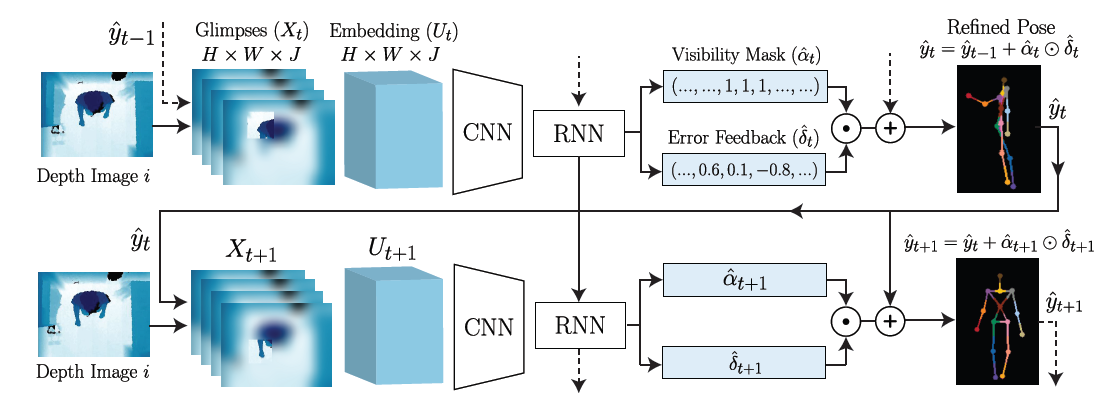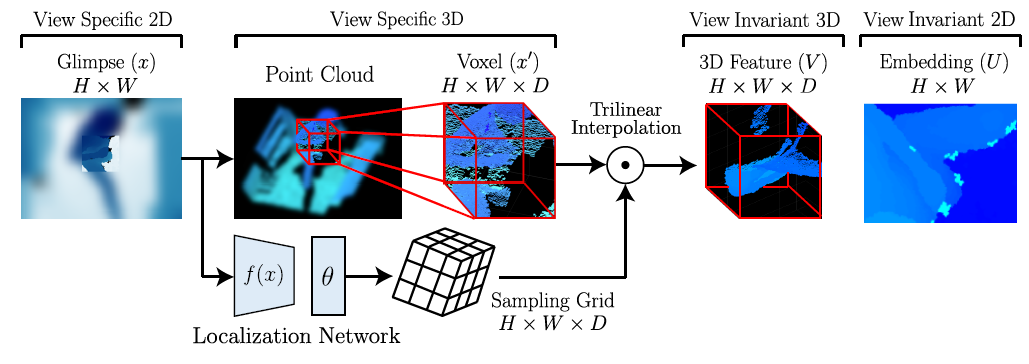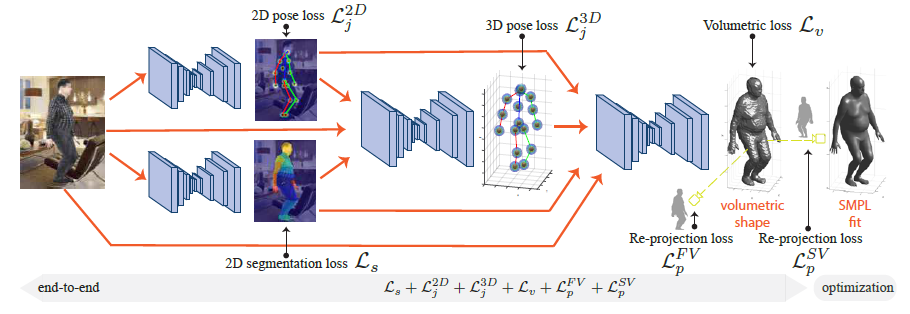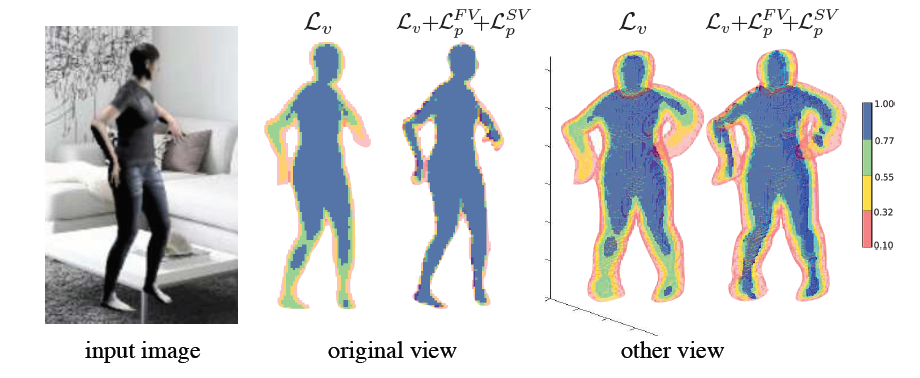# 关于3D姿态估测的论文阅读笔记

##  [ECCV2016] Towards Viewpoint Invariant 3D Human Pose Estimation- Local Input Representation

Glimpse: $X\in \mathbb{R}^{H\times W\times J}$, 其中$H$和$W$表示glimpse图像的大小，$J$是关节点的数目。论文里将glimpse图像比喻成视网膜，它是一种中心较清晰，边缘较模糊的图像编码方式。这让中心的特征可以被focus，同时能够保留部分空间信息。

- Learned Viewpoint Invariant Embedding$$V_{ijk}=\sum_{a=1}^{H}\sum_{b=1}^{W}\sum_{c=1}^{D}x’_{abc}\mathrm{ker}\left(\frac{a-x_{ijk}^{(G)}}{H}\right)\mathrm{ker}\left(\frac{b-y_{ijk}^{(G)}}{W}\right)\mathrm{ker}\left(\frac{c-z_{ijk}^{(G)}}{D}\right)$$

$$U_{ij}=\sum_{c=1}^DV_{ijc}$$

$V$是对glimpse的3D特征表达，$U$是$V$映射回二维空间的embedding结果。

- Convolutional and Recurrent Networks

##  [ECCV2018] BodyNet: Volumetric Inference of 3D Human Body Shapes- Volumetric Inference for 3D Human Shape

$V$表示要训练的网络占用图经过sigmoid激活后的值，$\hat{V}$是它的ground truth，$\mathcal{L}_v$定义为二者之间的交叉熵：

$$\mathcal{L}_v=\sum_{x=1}^W\sum_{y=1}^H\sum_{z=1}^DV_{xyz}\mathrm{log}\hat{V}_{xyz}+(1-V_{xyz})\mathrm{log}(1-\hat{V}_{xyz})$$

- Multi-view Re-projection Loss On the Silhouette$$\hat{S}^{FV}(x,y)=\max\limits_{z}\hat{V}_{xyz}$$

$$\hat{S}^{SV}(y,z)=\max\limits_{x}\hat{V}_{xyz}$$

$S^{FV}$和$S^{SV}$同理。$\mathcal{L}_p$依然定义为交叉熵：

$$\mathcal{L}_p^{FV}=\sum_{x=1}^W\sum_{y=1}^HS(x,y)\mathrm{log}\hat{S}^{FV}(x,y)+(1-S(x,y))\mathrm{log}(1-\hat{S}^{FV}(x,y))$$

$$\mathcal{L}_p^{SV}=\sum_{y=1}^H\sum_{z=1}^DS(y,z)\mathrm{log}\hat{S}^{SV}(y,z)+(1-S(y,z))\mathrm{log}(1-\hat{S}^{SV}(y,z))$$

- Multi-task Learning with Intermediate Supervision

2D姿态loss：用沙漏网络的前两层将$3\times256\times256$的RGB图像映射到$16\times64\times64$的2D关节热度图，其中$16$为关节数。这$16$张热度图是以每个关节为中心的高斯分布，放在一起取最大值就得到最终的2D关节定位热度图。$\mathcal{L}_{j}^{2D}$定义为关节定位热度图和ground truth之间的均方差。

2D分割loss：依然是用沙漏网络的前两层，将$3\times256\times256$的RGB图像映射到$15\times64\times64$的热度图，其中$15$为人体部位数。训练集基于一个叫做Skinned Multi-person Linear Model (SMPL)的模型。$\mathcal{L}_{s}$定义为人体部位定位热度图和ground truth之间的交叉熵。

3D姿态loss：将2D热度图延伸到3D，在体素网格上用3D的高斯分布表示3D关节定位。其中$xy$平面上就是2D的关节热度图，$z$轴上的值代表深度。实验表明，深度范围是一个大约85cm的区间，最多占用$19$位。所以最终的3D关节定位热度图大小为$64\times64\times19$。同样$\mathcal{L}_{j}^{3D}$定义为关节定位热度图和ground truth之间的均方差。

$$\mathcal{L}=\lambda_{j}^{2D}\mathcal{L}_{j}^{2D}+\lambda_{s}\mathcal{L}_{s}+\lambda_{j}^{3D}\mathcal{L}_{j}^{3D}+\lambda_{v}\mathcal{L}_{v}+\lambda_{p}^{FV}\mathcal{L}_{p}^{FV}+\lambda_{p}^{SV}\mathcal{L}_{p}^{SV}$$